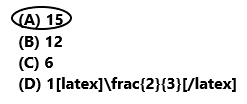# Texas Go Math Grade 5 Lesson 6.6 Answer Key Fraction and Whole-Number Division

Refer to our Texas Go Math Grade 5 Answer Key Pdf to score good marks in the exams. Test yourself by practicing the problems from Texas Go Math Grade 5 Lesson 6.6 Answer Key Fraction and Whole-Number Division.

## Texas Go Math Grade 5 Lesson 6.6 Answer Key Fraction and Whole-Number Division

Unlock the Problem

Three friends share a $$\frac{1}{4}$$-pound block of fudge equally. What fraction of a pound of fudge does each friend get?

Divide, $$\frac{1}{4}$$ ÷ 3
Let the rectangle represent a 1-pound block of fudge.
Divide the rectangle into fourths and then divide each fourth into three equal parts.
The rectangle is now divided into ___________ equals parts.When you divide one fourth into 3 equal parts, you are finding one of three equal parts or $$\frac{1}{3}$$ of $$\frac{1}{4}$$. Shade $$\frac{1}{3}$$ of $$\frac{1}{4}$$.
The shaded part is ___________ of the whole rectangle.
So, each friend gets ____________ of a pound of fudge.
Divide, 1/4 ÷ 3
Let the rectangle represent a 1-pound block of fudge.
Divide the rectangle into fourths and then divide each fourth into three equal parts.
The rectangle is now divided into 12 equals parts.When we divide one fourth into 3 equal parts, we are finding one of three equal parts or 1/3 of 1/4. Shade 1/3 of 1/4.
The shaded part is 1/12 of the whole rectangle.
So, each friend gets 1/12  of a pound of fudge.

Example

Brad has 9 pounds of ground turkey to make turkey burgers for a picnic. How many $$\frac{1}{3}$$-pound turkey burgers can he make?

Divide. 9 ÷ $$\frac{1}{3}$$
Draw 9 rectangles to represent each pound of ground turkey. Divide each rectangle into thirds.
When you divide the _________ rectangles into thirds, you are finding the number of thirds in 9 rectangles or finding 9 groups of _______. There are ________ thirds.Complete the number sentence.
So, Brad can make ________ one-third-pound turkey burgers.
Divide. 9 ÷ 1/3
Draw 9 rectangles to represent each pound of ground turkey. Divide each rectangle into thirds.
When we divide the 9 rectangles into thirds, we are finding the number of thirds in 9 rectangles or finding 9 groups of 3. There are 27 thirds.Complete the number sentence.
9 ÷ 1/3 = 9 x 3 = 27
So, Brad can make 27 one-third-pound turkey burgers.

Math Talk
Mathematical Processes

Will the number of turkey burgers be less than or greater than 9? Explain.
The number of turkey burgers are greater than 9.

Share and Show

Question 1.
Use the model to complete the number sentence.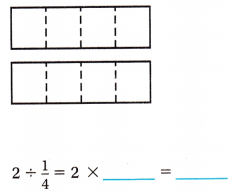Explanation:
In the above image we can observe 2 rectangles. Divide 2 ÷ 1/4. Draw 2 rectangles and divide each rectangle into fourths. When we divide the 2 rectangles into fourths, we are finding the number of fourths in 2 rectangles or finding 2 groups of 4. There are 8 fourths.
Question 2.
Use the model to divide.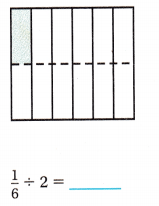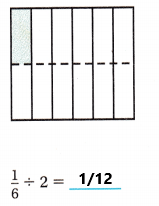Explanation:
In the above image we can observe 12 blocks. Divide, 1/6 ÷ 2. Divide the rectangle into sixths and then divide each sixths into two equal parts. The rectangle is now divided into 12 equals parts. When we divide one sixth into two equal parts, we are finding one of two equal parts or 1/2 of 1/6. Shaded 1/2 of 1/6. The shaded part is 1/12 of the whole rectangle.

Problem Solving

Divide.

Question 3.
5 ÷ $$\frac{1}{3}$$
5 ÷ 1/3 = 5 x 3 = 15
Explanation:
In the above division problem 5 ÷ 1/3 we can observe that the whole number is divided by a fraction. Divide 5 by 1/3 the result is 15. The result 15 is obtained by multiplying 5 with 3.

Question 4.
8 ÷ $$\frac{1}{2}$$
8 ÷ 1/2 = 8 x 2 = 16
Explanation:
In the above division problem 8 ÷ 1/2 we can observe that the whole number is divided by a fraction. Divide 8 by 1/2 the result is 16. The result 16 is obtained by multiplying 8 with 2.

Question 5.
$$\frac{1}{7}$$ ÷ 4
1/7 ÷ 4 = 1/7 x 1/4 = 1/28
Explanation:
In the above division problem 1/7 ÷ 4 we can observe that the fraction is divided by a whole number. Divide 1/7 by 4 the result is 1/28. The result 1/28 is obtained by multiplying 1/7 with 1/4.

Question 6.
$$\frac{1}{3}$$ ÷ 4
1/3 ÷ 4 = 1/3 x 1/4 = 1/12
Explanation:
In the above division problem 1/3 ÷ 4 we can observe that the fraction is divided by a whole number. Divide 1/3 by 4 the result is 1/12. The result 1/12 is obtained by multiplying 1/3 with 1/4.

Question 7.
$$\frac{1}{4}$$ ÷ 12
1/4 ÷ 12 = 1/4 x 1/12 = 1/48
Explanation:
In the above division problem 1/4 ÷ 12 we can observe that the fraction is divided by a whole number. Divide 1/4 by 12 the result is 1/48. The result 1/48 is obtained by multiplying 1/4 with 1/12.

Question 8.
6 ÷ $$\frac{1}{5}$$
6 ÷ 1/5 = 6 x 5 = 30
Explanation:
In the above division problem 6 ÷ 1/5 we can observe that the whole number is divided by a fraction. Divide 6 by 1/5 the result is 30. The result 30 is obtained by multiplying 6 with 5.

Question 9.
Write Math Communicate Describe how the model shows that dividing by $$\frac{1}{2}$$ is the same as multiplying by 2.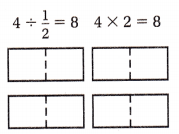The model shows that there are 8 halves in 4 wholes, and it shows that 4 groups of 2 is 8.
Explanation:
In the above image we can observe that whole number is divided by a fraction. If we divide 4 by 1/2 then the result is 8. Because when we multiply 8 with 1/2 the product is 4. So, dividing by 1/2 is same as multiplying by 2.

Question 10.
Describe how the model shows that dividing by 2 is the same as finding $$\frac{1}{2}$$ of $$\frac{1}{4}$$.The model shows that 1/4 divided into 2 equal groups is 1/8, and it shows that 1/2 of 1/4 is 1/8.
Explanation:
In the above image we can observe 8 blocks. Divide, 1/4 ÷ 2. Divide the rectangle into fourths and then divide each fourths into two equal parts. The rectangle is now divided into 8 equals parts. When we divide one fourth into two equal parts, we are finding one of two equal parts or 1/2 of 1/4. Shaded 1/2 of 1/4. The shaded part is 1/8 of the whole rectangle.

Unlock the Problem

Question 11.
Multi-Step The slowest mammal is the three-toed sloth. The top speed of a three-toed sloth on the ground is about $$\frac{1}{4}$$ foot per second. The top speed of a giant tortoise on the ground is about $$\frac{1}{3}$$ foot per second. How much longer would it take a three-toed sloth than a giant tortoise to travel 10 feet on the ground?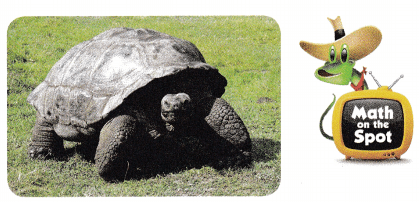(A) 10 seconds
(B) 30 seconds
(C) 40 seconds
(D) 70 seconds

a. What do you need to find?

b. What operations will you use to solve the problem?

c. Show the steps you used to solve the problem.

d. Complete the sentences.
A three-toed sloth would travel 10 feet in __________ seconds.
A giant tortoise would travel 10 feet in __________ seconds.
Since _______ – _________ = _________.
it would take a three-toed sloth _________ seconds longer to travel 10 feet.

e. Fill in the bubble for the correct answer choice.

Question 12.
Robert divides 8 cups of almonds into $$\frac{1}{8}$$-cup servings. How many servings does he have?
8 ÷ 1/8 = 8 x 8 = 64
He have 64 servings.
Explanation:
Robert divides 8 cups of almonds into 1/8-cup servings. In this division problem 8 ÷ 1/8 we can observe that the whole number is divided by a fraction. Divide 8 by 1/8 the result is 64. The result 64 is obtained by multiplying 8 with 8. So, Robert have 64 servings.

Question 13.
Your friend asks you to watch his iguana. He gives you 1/3 pound of food for 4 days. He tells you if you feed the iguana the same amount each day, you will have no food left over. How much food should you feed the iguana each day?
(A) $$\frac{1}{7}$$ pound
(B) 7 pounds
(C) 12 pounds
(D) $$\frac{1}{12}$$ pound

Question 14.
Representations lake modeled a division problem. Which problem does his model represent?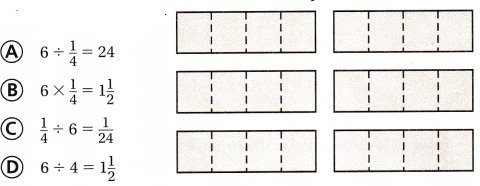The number sentence is 6 ÷ 1/4 = 6 x 4 = 24
So, Option A is correct.
Explanation:
Divide 6 ÷ 1/4. Draw 6 rectangles and divide each rectangle into fourths. When we divide the 6 rectangles into fourths, we are finding the number of fourths in 6 rectangles or finding 6 groups of 4. There are 24 fourths.
The problem 6 ÷ 1/4 represents the above model. So, draw a circle for option A.

Question 15.
Multi-Step Five people are going to share $$\frac{1}{4}$$ gallon of juice. After the juice is poured equally, Sheila gives her glass to Maria. How much juice does Maria have?
(A) $$\frac{1}{40}$$ gal
(B) $$\frac{4}{16}$$ gal
(C) $$\frac{1}{10}$$ gal
(D) $$\frac{1}{20}$$ gal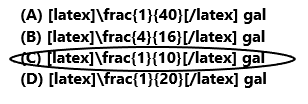1/4 ÷ 5 = 1/20
1/20 + 1/20 = 2/20 = 1/10
Maria have 1/10 gallon of juice.
So, option C is correct.
Explanation:
Five people are going to share 1/4 gallon of juice. The juice is poured equally. divide 1/4 by 5 the result is 1/20. After the juice is poured equally. Sheila gives her glass to Maria. Add 1/20 with 1/20 the sum is 1/10. So, Maria have 1/10 gallon of juice. Draw a circle for option C.

Texas Test Prep

Question 16.
Tina cuts $$\frac{1}{3}$$ yard of fabric into 4 equal parts. What is the length of each part?
(A) 12 yards
(B) 1$$\frac{1}{3}$$ yards
(C) 3$$\frac{2}{3}$$ yards
(D) $$\frac{1}{12}$$ yard1/3 ÷ 4 = 1/3 x 1/4 = 1/12
The length of each part is 1/12.
So, option D is correct.
Explanation:
Tina cuts 1/3 yard of fabric into 4 equal parts. Divide 1/3 by 4 the result is 1/12. The result 1/12 is obtained by multiplying 1/3 with 1/4. The length of each part is 1/12. So, draw a circle for option D.

### Texas Go Math Grade 5 Lesson 6.6 Homework and Practice Answer Key

Divide.

Question 1.
6 ÷ $$\frac{1}{3}$$
6 ÷ 1/3 = 6 x 3 = 18
Explanation:
In the above division problem 6 ÷ 1/3 we can observe that the whole number is divided by a fraction. Divide 6 by 1/3 the result is 18. The result 18 is obtained by multiplying 6 with 3.

Question 2.
4 ÷ $$\frac{1}{8}$$
4 ÷ 1/8 = 4 x 8 = 32
Explanation:
In the above division problem 4 ÷ 1/8 we can observe that the whole number is divided by a fraction. Divide 4 by 1/8 the result is 32. The result 32 is obtained by multiplying 4 with 8.

Question 3.
$$\frac{1}{3}$$ ÷ 9
1/3 ÷ 9 = 1/3 x 1/9 = 1/27
Explanation:
In the above division problem 1/3 ÷ 9 we can observe that the fraction is divided by a whole number. Divide 1/3 by 9 the result is 1/27. The result 1/27 is obtained by multiplying 1/3 with 1/9.

Question 4.
7 ÷ $$\frac{1}{2}$$
7 ÷ 1/2 = 7 x 2 = 14
Explanation:
In the above division problem 7 ÷ 1/2 we can observe that the whole number is divided by a fraction. Divide 7 by 1/2 the result is 14. The result 14 is obtained by multiplying 7 with 2.

Question 5.
8 ÷ $$\frac{1}{3}$$
8 ÷ 1/3 = 8 x 3 = 24
Explanation:
In the above division problem 8 ÷ 1/3 we can observe that the whole number is divided by a fraction. Divide 8 by 1/3 the result is 24. The result 24 is obtained by multiplying 8 with 3.

Question 6.
$$\frac{1}{4}$$ ÷ 4
1/4 ÷ 4 = 1/4 x 1/4 = 1/16
Explanation:
In the above division problem 1/4 ÷ 4 we can observe that the fraction is divided by a whole number. Divide 1/4 by 4 the result is 1/16. The result 1/16 is obtained by multiplying 1/4 with 1/4.

Question 7.
9 ÷ $$\frac{1}{4}$$
9 ÷ 1/4 = 9 x 4 = 36
Explanation:
In the above division problem 9 ÷ 1/4 we can observe that the whole number is divided by a fraction. Divide 9 by 1/4 the result is 36. The result 36 is obtained by multiplying 9 with 4.

Question 8.
$$\frac{1}{7}$$ ÷ 2
1/7 ÷ 2 = 1/7 x 1/2 = 1/14
Explanation:
In the above division problem 1/7 ÷ 2 we can observe that the fraction is divided by a whole number. Divide 1/7 by 2 the result is 1/14. The result 1/14 is obtained by multiplying 1/7 with 1/2.

Question 9.
10 ÷ $$\frac{1}{5}$$
10 ÷ 1/5 = 10 x 5 = 50
Explanation:
In the above division problem 10 ÷ 1/5 we can observe that the whole number is divided by a fraction. Divide 10 by 1/5 the result is 50. The result 50 is obtained by multiplying 10 with 5.

Question 10.
5 ÷ $$\frac{1}{5}$$
5 ÷ 1/5 = 5 x 5 = 25
Explanation:
In the above division problem 5 ÷ 1/5 we can observe that the whole number is divided by a fraction. Divide 5 by 1/5 the result is 25. The result 25 is obtained by multiplying 5 with 5.

Question 11.
$$\frac{1}{5}$$ ÷ 12
1/5 ÷ 12 = 1/5 x 1/12 = 1/60
Explanation:
In the above division problem 1/5 ÷ 12 we can observe that the fraction is divided by a whole number. Divide 1/5 by 12 the result is 1/60 The result 1/60 is obtained by multiplying 1/5 with 1/12.

Question 12.
$$\frac{1}{9}$$ ÷ 5
1/9 ÷ 5 = 1/9 x 1/5 = 1/45
Explanation:
In the above division problem 1/9 ÷ 5 we can observe that the fraction is divided by a whole number. Divide 1/9 by 5 the result is 1/45. The result 1/45 is obtained by multiplying 1/9 with 1/5.

Problem Solving

Question 13.
Cooks at a food booth at the state fair made an extra-large 8-foot long burrito. If they cut the burrito into $$\frac{1}{4}$$-foot servings, how many people will the extra-large burrito serve?
8 ÷ 1/4 = 8 x 4 = 32
The extra-large burrito is served by 32 people.
Explanation:
Cooks at a food booth at the state fair made an extra-large 8-foot long burrito. They cut the burrito into 1/4-foot servings. Divide 8 by 1/4 the result is 32. So, the extra-large burrito is served by 32 people.

Question 14.
A serving of the burrito contains $$\frac{1}{4}$$ pound of meat. Franklin and two friends share his serving equally. How much meat does each friend get?
1/4 ÷ 3 = 1/12
Each friend get 1/12 meat.
Explanation;
A serving of the burrito contains 1/4 pound of meat. Franklin and two friends share his serving equally. Divide 1/4 by 3 the result is 1/12.

Lesson Check

Question 15.
Toby used the model below to help him divide.Which problem was he trying to solve?
(A) $$\frac{1}{6}$$ ÷ 2
(B) 2 ÷ 6
(C) 2 ÷ $$\frac{1}{6}$$
(D) 2 × $$\frac{1}{6}$$The number sentence is 2 ÷ 1/6 = 2 x 6 = 12
So, Option C is correct.
Explanation:
Divide 2 ÷ 1/6. Draw 2 rectangles and divide each rectangle into sixths. When we divide the 2 rectangles into sixths, we are finding the number of sixths in 2 rectangles or finding 2 groups of 6. There are 12 sixths.
The problem 2 ÷ 1/6 represents the above model. So, draw a circle for option C.

Question 16.
Which model shows the solution for $$\frac{1}{2}$$ ÷ 3?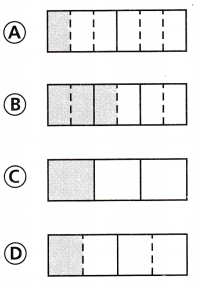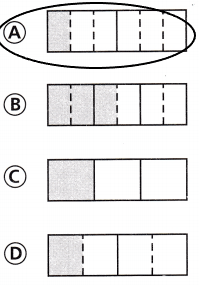1/2 ÷ 3 = 1/6
So, option A is correct.
Explanation:
Divide, 1/2 ÷ 3. Divide the rectangle into 1/2 and then divide each 1/2 into three equal parts. The rectangle is now divided into 6 equals parts. When we divide 1/2 into two equal parts, we are finding one of two equal parts or 1/2 of 1/3.  The shaded part is 1/6 of the whole rectangle. So, draw a circle for option A.
Question 17.
Analise cuts $$\frac{1}{2}$$ yard of rope into 8 equal parts. What is the length of each part?
(A) $$\frac{1}{16}$$ yard
(B) $$\frac{1}{10}$$ yard
(C) $$\frac{1}{4}$$ yard
(D) $$\frac{1}{6}$$ yard1/2 ÷ 8 = 1/16
The length of each part is 1/16.
So, option A is correct.
Explanation:
Analise cuts 1/2 yard of rope into 8 equal parts. Divide 1/2 by 8 the result is 1/16. The result 1/16 is obtained by multiplying 1/2 with 1/8. So, draw a circle for option A.

Question 18.
The dragon costume for the new year celebration is 9 yards long. If Brittany sews a button on each $$\frac{1}{6}$$-yard section, how many buttons will she sew?
(A) 63
(B) 56
(C) 15
(D) 54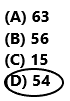9 ÷ 1/6 = 9 x 6 = 54
She sewed 54 buttons.
So, option D is correct.
Explanation:
The dragon costume for the new year celebration is 9 yards long. Brittany sews a button on each 1/6-yard section. Divide 9 by 1/6 the result is 54. The result 64 is obtained by multiplying 9 with 6. She sewed 54 buttons. So draw a circle for option D.

Question 19.
Multi-Step Ruby has a collection of 16 stuffed animals. She gives $$\frac{1}{4}$$ of her collection to her younger brother. Then she gives half of the remaining animals to her baby sister. How many animals does Ruby give her sister?
(A) 4
(B) 2
(C) 6
(D) 816 x (1/4) = 4
She gave 4 of her collections to her younger brother.
16 – 4 = 12
Now, Ruby have 12 animals.
12 ÷ 2 = 6
Ruby gave 6 animals to her sister.
So option C is correct.
Explanation:
Ruby has a collection of 16 stuffed animals. She give 1/4 of her collection to her younger brother. Multiply 16 with 1/4 the product is 4. She gave 4 of her collections to her younger brother. Subtract 4 from 16 the difference is 12. Then she gives half of the remaining animals to her baby sister. Divide 12 by 2 the result is 6. Ruby gave 6 animals to her sister. So draw a circle for option C.

Question 20.
Multi-Step Ahmad and Kiera are mixing paints for the students in their art class. They mix 2 pints of blue paint, 2 pints of white paint, and 1 pint of green paint. How many $$\frac{1}{3}$$-pint containers can they fill with the mixed paint?
(A) 15
(B) 12
(C) 6
(D) 1$$\frac{2}{3}$$